# Easiest colleges to get into in Georgia

Top 10 colleges in Georgia with the highest acceptance rates
What are the easiest colleges to get into in Georgia? We've got you covered. We've compiled a national college database and have created a list of the easiest colleges to get into in Georgia below. These are the easiest 4 year colleges to get into in Georgia that have the highest acceptance rates, and are more likely to accept low GPAs, GEDs, and everything in between. There are tons of good colleges below that accept more applicants, and we also share their average SAT and ACT scores so that you can see where you're most competitive. Read on to find out more.

## Life University acceptance rate

Life University acceptance rate is 99.3%.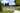The average SAT score for Life University is 970.

• SAT composite: 970
• SAT math: 480

The average ACT score for Life University is 19.

## Covenant College acceptance rate

Covenant College acceptance rate is 97.2%.The average SAT score for Covenant College is 1240.

• SAT composite: 1240
• SAT math: 600

The average ACT score for Covenant College is 27.

## Middle Georgia State University acceptance rate

Middle Georgia State University acceptance rate is 94%.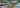The average SAT score for Middle Georgia State University is 935.

• SAT composite: 935
• SAT math: 460

The average ACT score for Middle Georgia State University is 19.

## Reinhardt University acceptance rate

Reinhardt University acceptance rate is 90%.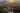The average SAT score for Reinhardt University is 1100.

• SAT composite: 1100
• SAT math: 540

The average ACT score for Reinhardt University is 21.

## Georgia Gwinnett College acceptance rate

Georgia Gwinnett College acceptance rate is 88%.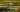The average SAT score for Georgia Gwinnett College is 1012.

• SAT composite: 1012
• SAT math: 500

The average ACT score for Georgia Gwinnett College is 19.

## Savannah State University acceptance rate

Savannah State University acceptance rate is 82.6%.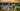The average SAT score for Savannah State University is 940.

• SAT composite: 940
• SAT math: 460

The average ACT score for Savannah State University is 17.

## Georgia College acceptance rate

Georgia College acceptance rate is 77.8%.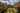The average SAT score for Georgia College is 1198.

• SAT composite: 1198
• SAT math: 590

The average ACT score for Georgia College is 25.

## Morehouse College acceptance rate

Morehouse College acceptance rate is 74.4%.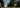The average SAT score for Morehouse College is 1058.

• SAT composite: 1058
• SAT math: 520

The average ACT score for Morehouse College is 21.

## Valdosta State University acceptance rate

Valdosta State University acceptance rate is 74.2%.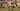The average SAT score for Valdosta State University is 1060.

• SAT composite: 1060
• SAT math: 520

The average ACT score for Valdosta State University is 21.

## Mercer University acceptance rate

Mercer University acceptance rate is 73.3%.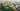The average SAT score for Mercer University is 1263.

• SAT composite: 1263
• SAT math: 630